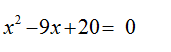# Solve the equation graphically in the given interval. State each answer rounded to two decimals. (Enter your answers as a comma-separated list.)x2 − 9x + 20 = 0;   [0, 7]

Question
1 views

Solve the equation graphically in the given interval. State each answer rounded to two decimals. (Enter your answers as a comma-separated list.)

x2 − 9x + 20 = 0;   [0, 7]
check_circle

Step 1

Given equation:Since the given equation is a quadratic equation and the graph of a quadratic function is a parabola.

Now,

The graph of the quadratic equation is:

...

### Want to see the full answer?

See Solution

#### Want to see this answer and more?

Solutions are written by subject experts who are available 24/7. Questions are typically answered within 1 hour.*

See Solution
*Response times may vary by subject and question.
Tagged in